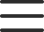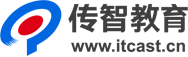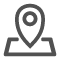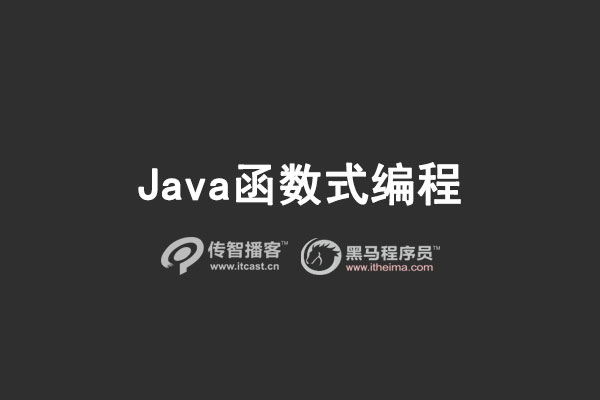# Java函数式编程入门教程[java培训]

1. 函数式编程简介

Java作为面向对象的编程语言，如果按照编程种类划分属于命令式编程(Imperative Programming)。常见的编程范式还有逻辑式编程(Logic Programming)，函数式编程(Functional Programming)。2. Lambda 表达式

Java 8的最大变化是引入了Lambda(Lambda 是希腊字母 λ 的英文名称)表达式——一种紧凑的、传递行为的方式。

2.1 Lambda 表达式的形式

lambda表达式的语法由参数列表、箭头符号->和函数体组成。函数体既可以是一个表达式，也可以是一个语句块。

return语句会把控制权交给匿名方法的调用者。

break和continue只能在循环中使用。

(int x, int y) ‐> x + y
() ‐> 42
(String s) ‐> { System.out.println(s); }

2.2 常见应用

2.2.1 替代匿名内部类

@Test
public void oldRunable() {
@Override
public void run() {
System.out.println("The old runable now is using!");
}
}).start();
}

@Test
public void runable() {
new Thread(() ‐> System.out.println("It's a lambda
function!")).start();
}

The old runable now is using!
It's a lambda function!

2.2.2 使用lambda表达式对集合进行迭代

Java的集合类是日常开发中经常用到的，甚至说没有哪个java代码中没有使用到集合类。。。而对集合类最常见的操作就是进行迭代遍历了。请看对比：

@Test
public void iterTest() {
List<String> languages = Arrays.asList("java","scala","python");
//before java8
for(String each:languages) {
System.out.println(each);
}
//after java8
languages.forEach(x ‐> System.out.println(x));
languages.forEach(System.out::println);
}

languages.forEach(System.out::println);

2.2.3 用lambda表达式实现map

@Test
public void mapTest() {
List<Double> cost = Arrays.asList(10.0, 20.0,30.0);
cost.stream().map(x ‐> x + x*0.05).forEach(x ‐>
System.out.println(x));
}

10.5

21.0

31.5

map函数可以说是函数式编程里最重要的一个方法了。map的作用是将一个对象变换为另外一个。在我们的例子中，就是通过map方法将cost增加了0.05倍的大小然后输出。

2.2.4 用lambda表达式实现map与reduce

@Test
public void mapReduceTest() {
List<Double> cost = Arrays.asList(10.0, 20.0,30.0);
double allCost = cost.stream().map(x ‐> x+x*0.05).reduce((sum,x)
‐> sum + x).get();
System.out.println(allCost);
}

63.0

@Test
public void sumTest() {
List<Double> cost = Arrays.asList(10.0, 20.0,30.0);
double sum = 0;
for(double each:cost) {
each += each * 0.05;
sum += each;
}
System.out.println(sum);
}

2.2.5 filter操作

filter也是我们经常使用的一个操作。在操作集合的时候，经常需要从原始的集合中过滤掉一部分元素。

@Test
public void filterTest() {
List<Double> cost = Arrays.asList(10.0, 20.0,30.0,40.0);
List<Double> filteredCost = cost.stream().filter(x ‐> x > 25.0).collect(Collectors.toList());
filteredCost.forEach(x ‐> System.out.println(x));
}

30.0

40.0

JVM内存模型详细介绍